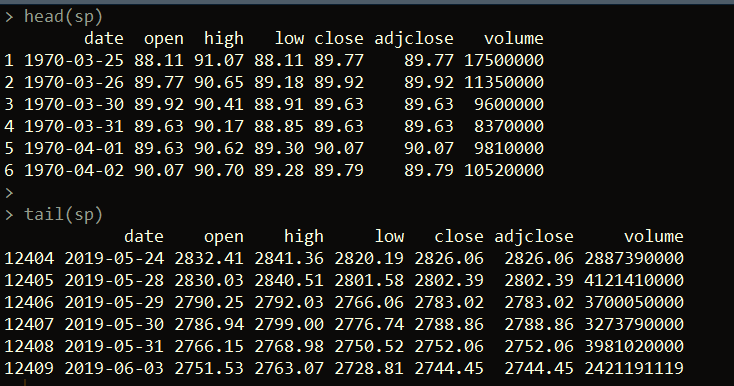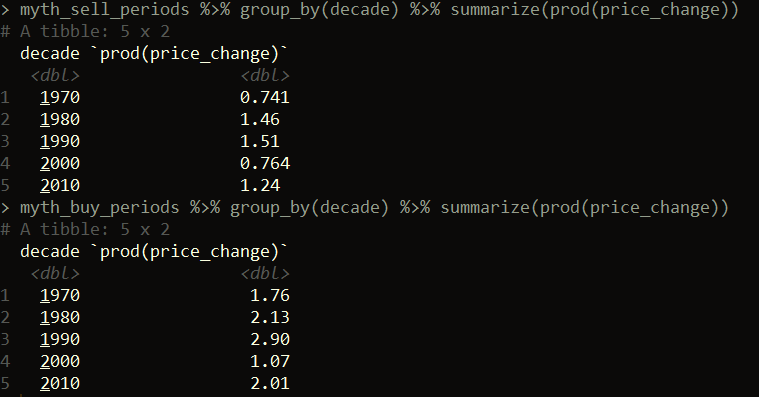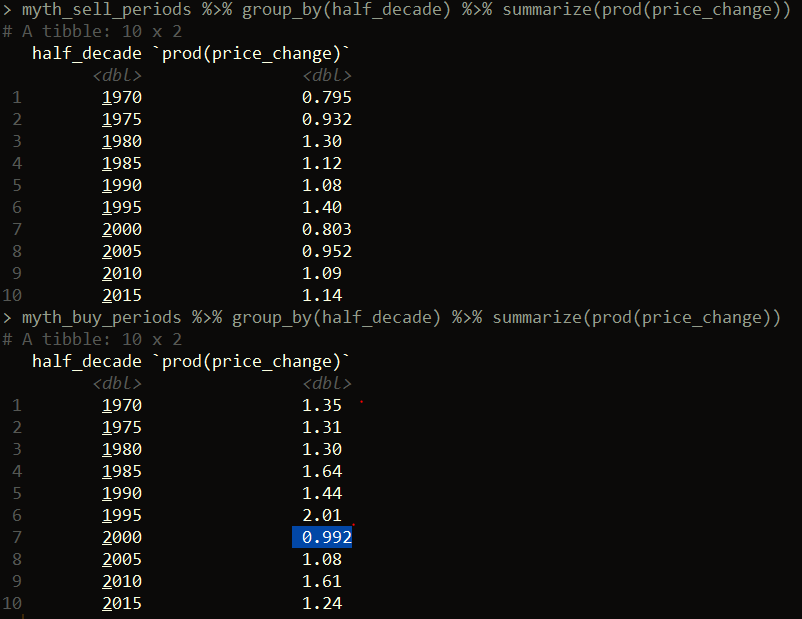Want to share your content on R-bloggers? click here if you have a blog, or here if you don't.If you follow the stock market, you’ve probably heard the expression “Sell in May, Go Away.” This expression generally refers to the perceived idea that the stock market goes up between the end of October and end of April, but one should sell at the beginning of May to avoid losses. The general recommendation according to the theory is to hold money in a money market account during the “short period” of May through October, and then reinvest in the stock market in November. But how does this myth hold up in reality? Let’s use R to find out! Our analysis will look strictly at the S&P 500 performance during the years 1970 to the present (so we won’t dive into interest rate levels, money market accounts, etc.).

## Getting started

```
library(dplyr)
library(chron)

sp <- read.csv("sp_data.csv", stringsAsFactors = FALSE)

```Next, let’s create a few fields that parse out the year, month, and day from the date column. We’ll use these shortly.

```
# convert date field to a Date type
sp\$date <- as.Date(sp\$date)

# Get month, year, and day using the chron package
sp\$month <- months(sp\$date)
sp\$year <- years(sp\$date)
sp\$day <- days(sp\$date)

```

## Getting end of month prices

Let’s break down exactly what we need to do now. What is it really that we’re testing in this theory? In effect, we need to calculate the price change between the end of April and end of October each year. This will show us what the performance of the S&P 500 was like in each year during the “short period.” We also need to calculate the price change between the end of October and the end of the following April, which we’ll call the “long period.”

This means that we need to get the closing prices of the S&P 500 at the end of every October and April. We can do this pretty easily with dplyr.

```
# get end-of-month prices
eom_prices <- sp %>% arrange(year, month, desc(day)) %>%
distinct(month, year, .keep_all = TRUE) %>%
arrange(date)

```The code above sorts the price data by descending order of day of the month for each month and year, and then takes the first occurring (using the distinct function) month-year record. Since we sorted in descending order by day for each month-year, this will give us the record of the last trading day in the month for each month-year combo. Figuring this out using dplyr allows us to avoid having to figure out the last trading day of each month by some other means e.g. using another package or importing data from elsewhere.

Next, we just need to filter our dataset down to just the months of April and October since all the end-of-month prices we need to compare come from these months.

```
only <- eom_prices %>% filter(month %in% c("April", "October"))

```

## Calculate the price change across each period

Now we just need to calculate the price changes between April and the subsequent October, and October and the subsequent April for each April / October. We’ll calculate the price change as the factor to multiply to get from one closing price to the next. So if the S&P went up by 5% over one of the periods, the price_change column value would be 1.05.

```

```

Next, let’s split our dataset into the buy or long periods vs. the sell / short periods.

```
myth_sell_periods <- only %>% filter(month == "April")
myth_buy_periods <- only %>% filter(month == "October")

nrow(myth_sell_periods) # 50

```

To make our analysis comparable, let’s make it so we’re looking at the same number of records for the short periods and long periods.

```
myth_sell_periods <- myth_sell_periods[1:49,]

```

If we take the product of the price_change column in each dataset, we can see how much we would multiply an initial investment in the long period would be worth if the investment was sold at the end of each long period and then re-bought at the start of the next. Similarly, we could do the same if we simulate buying at the start of each short period and selling at the end of each short period (and then re-buying at the start of the next short period).

## What’s the performance?

This analysis is not taking into account transaction fees, taxes, or inflation. However, from this we can see that the investments in the long periods over time would result in ~23x an initial principle. So \$100 would grow to ~\$2329.90 and \$100 invested only in the short periods would grow to ~\$155.1. This means over the full period from 1970 to the present, an investor would have made money in both the short (**though accounting for inflation, the short periods are not actually positive) and long periods – though the performance is much (on the surface) better during the long periods.

```

prod(myth_sell_periods\$price_change) # 1.550979

```

Let’s dig further into this. One point to note is that the brunt of the 2008 stock market crash happened during a short period. If we filter our data to look at everything prior to 2008, we get a similar, but less extreme, picture.

```
myth_buy_periods %>% filter(year < 2008) %>%
select(price_change) %>% prod() # 11.26064

myth_sell_periods %>% filter(year < 2008) %>%
select(price_change) %>% prod() # 1.509411

```

Now, let’s examine instance by instance, how many times the short period had a positive return versus the long period.

```
# 38
myth_buy_periods %>% filter(price_change > 1) %>% nrow()

# 33
myth_sell_periods %>% filter(price_change > 1) %>% nrow()

```

From this, we can can see that the long period had positive returns 38 out of 49 times. The short period had positive returns 33 out of 49 times.

We can also look at the decade-by-decade performance of the long and short periods.

```

```Here we can see the overall decade performance of the long periods was positive for each decade, whereas for the short periods, it was negative in the 1970’s and 2000’s. Let’s narrow our analysis down to half-decade periods.

```
myth_sell_periods\$half_decade <- floor(as.numeric(as.character(myth_sell_periods\$year)) / 5) * 5

```As you can see by the highlighted record, there’s one 5-year period where the performance was negative (2000 – 2004), but only slightly. Every other 5-year period had a positive return for the long periods. The short periods had negative returns in four periods (1970 – 1974, 1975 – 1979, 2000 – 2004, and 2005 – 2009).

In summary, the performance during the long periods (end of October through end of April) seems to be better on the surface than performance during the short periods (end of April through end of October). If you look back historically, part of the reason driving this has to do with significant economic events having a large effect on the stock market during the short periods e.g. the stock market decline in September – October 2008. Like anything in investing, past performance is no guarantee of future performance, so do with that knowledge what you will. There are always other factors to consider e.g. comparisons between multiple investment options, taxes, etc.

• Getting data from PDFs the easy way with R
• Those “other” apply functions: rapply, vapply, and eapply
• How to run R from the Task Scheduler
• The post Does “Sell in May, Go Away” really work? appeared first on Open Source Automation.